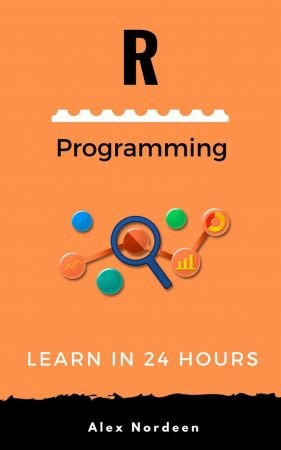Article info
• Views: 0
• Author: rukensai
• Date: 17-11-2021, 21:11
17-11-2021, 21:11

### Learn R Programming in 24 Hours by Alex Nordeen

Category: E-BooksEnglish | 2021 | ISBN: 1230004365112 | 681 Pages | EPUB | 4 MB

R is a programming language developed is widely used for statistical and graphical analysis. It can execute advance machine learning algorithms including earning algorithm, linear regression, time series, statistical inference.
R programming language is used by Fortune 500 companies and tech bellwethers like Uber, Google, Airbnb, Facebook, Apple.

R provides a data scientist tools and libraries (Dplyr) to perform the 3 steps of analysis 1) Extract 2) Transform, Cleanse 3) Analyze.

Chapter 1: What is R Programming Language? Introduction & Basics

Chapter 2: How to Download & Install R, RStudio, Anaconda on Mac or Windows

Chapter 3: R Data Types, Arithmetic & Logical Operators with Example

Chapter 4: R Matrix Tutorial: Create, Print, add Column, Slice

Chapter 5: Factor in R: Categorical & Continuous Variables

Chapter 6: R Data Frame: Create, Append, Select, Subset

Chapter 7: List in R: Create, Select Elements with Example

Chapter 8: R Sort a Data Frame using Order()

Chapter 9: R Dplyr Tutorial: Data Manipulation(Join) & Cleaning(Spread)

Chapter 10: Merge Data Frames in R: Full and Partial Match

Chapter 11: Functions in R Programming (with Example)

Chapter 12: IF, ELSE, ELSE IF Statement in R

Chapter 13: For Loop in R with Examples for List and Matrix

Chapter 14: While Loop in R with Example

Chapter 15: apply(), lapply(), sapply(), tapply() Function in R with Examples

Chapter 16: Import Data into R: Read CSV, Excel, SPSS, Stata, SAS Files

Chapter 17: How to Replace Missing Values(NA) in R: na.omit & na.rm

Chapter 18: R Exporting Data to Excel, CSV, SAS, STATA, Text File

Chapter 19: Correlation in R: Pearson & Spearman with Matrix Example

Chapter 20: R Aggregate Function: Summarise & Group_by() Example

Chapter 21: R Select(), Filter(), Arrange(), Pipeline with Example

Chapter 22: Scatter Plot in R using ggplot2 (with Example)

Chapter 23: How to make Boxplot in R (with EXAMPLE)

Chapter 24: Bar Chart & Histogram in R (with Example)

Chapter 25: T Test in R: One Sample and Paired (with Example)

Chapter 26: R ANOVA Tutorial: One way & Two way (with Examples)

Chapter 27: R Simple, Multiple Linear and Stepwise Regression [with Example]

Chapter 28: Decision Tree in R with Example

Chapter 29: R Random Forest Tutorial with Example

Chapter 30: Generalized Linear Model (GLM) in R with Example

Chapter 31: K-means Clustering in R with Example

Chapter 32: R Vs Python: What's the Difference?

Chapter 33: SAS vs R: What's the Difference?